Reach Us+44-1522-440391
Comparison of the Count Regression Models in Evaluation of the Effects of Hazelnut Harvest Season Variations on Pulmonary Aspergillus | OMICS International
Journal of Biometrics & Biostatistics

Like us on:

All submissions of the EM system will be redirected to Online Manuscript Submission System. Authors are requested to submit articles directly to Online Manuscript Submission System of respective journal.

# Comparison of the Count Regression Models in Evaluation of the Effects of Hazelnut Harvest Season Variations on Pulmonary Aspergillus

Esin A1* and Emel U2

1Department of Applied Statistics, Giresun University, Giresun, Turkey

2Department of Medical Microbiology, Giresun University, Giresun, Turkey

*Corresponding Author:
Esin A
Faculty of Art and Science
Department of Applied Statistics
Giresun University, Giresun, Turkey
Tel: +90 454 310 1000
E-mail: [email protected]

Received Date: November 30, 2015; Accepted Date: January 02, 2016; Published Date: January 16, 2016

Citation: Esin A, Emel U (2016) Comparison of the Count Regression Models in Evaluation of the Effects of Hazelnut Harvest Season Variations on Pulmonary Aspergillus. J Biom Biostat 7:278. doi:10.4172/2155-6180.1000278

Copyright: © 2016 Esin A, et al. This is an open-access article distributed under the terms of the Creative Commons Attribution License, which permits unrestricted use, distribution, and reproduction in any medium, provided the original author and source are credited.

Visit for more related articles at Journal of Biometrics & Biostatistics

#### Abstract

Pulmonary aspergillosis has recently emerged as a worldwide health care problem especially in patients with underlying lung disease. The objective of this study was to compare the Poisson and COM-Poisson regression models and to find the best fitted model for determining the effect of hazelnut harvest season on pulmoner aspergillosis. The data obtained from the state hospital of our cityin the time period of two years, from September, 2012 to August, 2014. A retrospective study was conducted. Respiratory specimens which showed repeated isolation of Aspergillus were included in the study however only one of the samples was analysed. Cases were classified according to revised definitions given by European Organization for Research and Treatment of Cancer/Invasive Mycosis Study Consensus Group (EORT/MSG). Culture positive 36 patients were detected from 3457 patients. Poisson and Conway-Maxwell- Poisson (COM-Poisson) regression models were compared to determine the best fitted model for identifying the number of new pulmonary aspergillosis cases in hazelnut harvest season. To describe the best fitted model of count data, dispersion, deviance and Akaike Information Criteria (AIC) test statistics were used. Based on statistical test for dispersion, the under-dispersion was found non-significant. This results clearly indicate that Poisson regression model is more approtiate for pulmonary aspergillosis data when compared to COM-Poisson regression model. Deviance and AIC values also confirm this result. Poisson regression model and COM- Poisson regression model were compared with statistical tests. According to statistical tests Poisson regression model was found to be the best fit model for pulmonary aspergillosis data.

#### Keywords

AIC; Poisson regression; COM-Poisson regression; Pulmonary aspergillosis; Hazelnut harvest season.

#### Introduction

Pulmonary aspergillosis has recently emerged as a worldwide health care problem especially in patients with underlying lung disease. Aspergillus spp. is a saprophytic and pathogenic fungus with a cosmopolitan distiribution. It is best known for its colonisation of tree nuts. Aspergillus infections can occur while hosts are still in the field, but often show no symptoms until postharvest storage and/or transport [1,2].

In Turkey on Black Sea Cost, hazelnuts are harvested annually in late August and September. During the harvest season spores of fungi disperse with the wind and many COPD and asthma patients apply to the hospital with exacerbation of the disease secondary to infection following the harvest. These exacerbations cause extensive use of broad spectrum antibiotics, immunosuppressive agents and increasing population of terminally ill patients.

In our study the data obtained from a state hospital in the time period of two years, from September, 2012 to August, 2014. A retrospective study was conducted. Demographic details, clinical and radiological findings, predisposing factors and treatments were noted down. Cases were classified as proven, probable and possible invasive Aspergillosis according to revised definitions given by European Organization for Research and Treatment of Cancer/Invasive Mycosis Study Consensus Group (EORT/MSG) . According to the suggestion of this group, all culture positive patients and those fullfilling the following clinical criteria were included in the study; 1) Long term sterioid therapy 2) radiological features suggestive of Aspergillosis 3) Microbiological findings. To rule out the changes of contamination respiratory specimens which showed repeated isolation of Aspergillus were included in the study however only one of the sample taken for analysis. To demonstrate the relationship with hazelnutfarm harvest season (August and September), number of the patients were divided into two part according to their hospital visits whether in the nuts picking season or not. Culture positive 36 patients were detected.

All samples were identified as Aspergillus spp. morphologically. Patients were on steroid treatment at a minimum dose of 0.3 mg/kg/ day of prednisone equivalent for more than three weeks. They had at least one radiological findings supporting the diagnosis of pulmonary aspergillosis. Despite the antifungal therapy 22.2% of the patients died. In clinical work the researcher often encounters situations where the outcome variable is numeric, but in the form of counts. Often it is a count of rare events for a certain period of time. To explore the relationship between dependent variable and some or all of the explanatory variables regression analysis are used . If the dependent variable characterized by rare events count data and all the counts are positive integers Poisson distribution is the most common for fitting. The main assumption of Poisson distribution is that the mean and variance are equal (equidispersion). Unfortunately many real data do not adhere this assumption . In case of violation of assumption, two-parameter generalized form of the Poisson distribution, called the Conway-Maxwell-Poisson (COM-Poisson) distribution allows for over (i.e., the variance is larger than the mean)-and under (i.e., the variance is smaller than the mean) dispersion .

In this study, we compared the Poisson to COM-Poisson regression model and illustrate its usefulness by using two years period of pulmonary aspergillosis data.

Poisson regression models

Poisson regression is one of the members of the Generalized Linear Models (GLM) framework. The simplest distribution used for modeling count data is the Poisson distribution with probability density function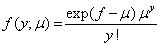(1)

The canonical link is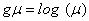resulting in a log-linear relationship between mean and linear predictor. The variance in the Poisson model is identical to the mean, thus the dispersion is fixed at v = 1 and the variance function is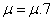. The mean Poisson regression can be assumed to follow a log link,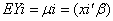, where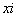denotes the vector of explanatory variables and β the vector of regression parameters. The maximum likelihood estimates can be obtained by maximizing the log likelihood.

Conway-Maxwell-Poisson (Com-Poisson) models

the Conway-Maxwell-Poisson (COM-Poisson) distribution has been re- introduced by statisticians to model count data characterized by either over- or under- dispersion [6-10]. The COM-Poisson distribution was first introduced in 1962 by Conway and Maxwell; in 2008 Guikema et al.  evaluated it in the context of a GLM, in the same year Bayesian approach of COM-Poisson are exteneded by Lord et al. . Sellers and Shmueli  enlarged the application area by developing COM Poisson Reg code in R package program. The COMPoisson distribution is a two-parameter generalization of the Poisson distribution that is flexible enough to describe a wide range of count data distributions .

The COM-Poisson probability distribution function is given by the equation: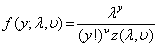(2)

for a random variable Y, where Z(λ,v) = and v ≥ 0 is a normalizing constant; v is considered the dispersion parameter such that v > 1 represents under-dispersion, and v < 1 over-dispersion. The COMPoisson distribution includes three well-known distribution as special cases: Poisson (v = 1), Geometric (v = 0,< 1), and Bernoulli well-known distribution as special cases: Poisson (v = 1), Geometric (v = 0, λ < 1),

Taking a GLM approach, Sellers and Shmueli (2010) proposed a COM-Poisson regression model using the link function,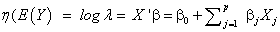(3)

Because of indirectly relationship between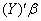, estimating β and v via associated normal equations become complex. Using (0) and (0) = 1, as starting values. These equations can thus be solved via an appropriate iterative reweighted least squares procedure (or by maximize ing the likelihood function directly using anoptimization program) to determine the maximum likelihood estimates,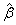and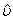. The associated standart errors of the estimated coefficients are derived using the Fisher Information matrix .

Testing for variable dispersion

In statistical analysis of count data, if the variance of the random variable is constrained to equal the mean which is called equidispersion, the Poisson regression model is usually adequate. Because of different factors can invalidate equidispersion hypothesis in the data its implicit restriction: consequently, data often exhibit overdispersion (i.e., the variance is larger than the mean) and, occasionally they exhibit underdispersion whith the mean exceeding the variance .

In GLM to detect over or under-dispersion simply, the researcher may look at the rule of thumb that the mean deviance, that is deviance/ degree of freedom should be close to unity. Deviance theoretically allows one to determine if the fitted GLM model is significantly worse than the saturated model .

Sellers et al.  established a hypothesis testing procedure to demonstarting the need for a COM-Poisson regression model over a simple Poisson regression model by determine if significant data dispersion exists or not, in other words, they test whether (v = 1) or otherwise . The test statistics,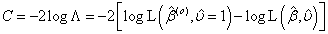(4)

where Λ is the likelihood ratio test statistic, β 0 are the maximum likelihood estimates obtained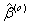under : v = 1 (i.e., the Poisson estimates), and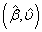, v are the maximum likelihood estimates under the general state space for the COM-Poisson distribution with 1 degree of freedom. For small samples, the test statistic distribution can be estimated via bootstarp .

Akaike information criteria (AIC)

In statistical literatures, based on several likelihood measure, one can compare several models performance. One of the most regularly used measure is AIC. The AIC penalized a model with larger number of parameters, and is defined as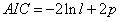(5)

where lnL denotes the fitted log likelihood and p the number of parameters . A relatively small value of AIC is prefered for the fitted model. Analyses were performed using R program. Respectievely, glm () function from "stats" and cmp() function from "COMPoissonReg" package were used.

#### Result and Conclusion

To obtain the first overview of the dependent variable, the histogram of the observed count frequencies were employed. Figure 1 illustrates the histogram, the marjinal distribution exhibits substantial variation (Figures 1 and 2).

Figure 2 presents line plot of total number of patients with positive culture. From the plot we could conclude that, especially in August and September the number of patients with positive culture were increased.

Poisson regression model was fitted to the data, regressing the number of patients with positive culture (Y) on hazelnutfarm harvest season (X) (1: Yes, 0: No); the estimated coefficients were given by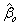= −0.1054, β1 = 1.6094, then COM- Poisson regression model was fitted to determine whether equi-dispersion was a reasonable assumption. After dividing the COM-Poisson coefficients by v dispersion parameter (-2.1822/1.5082 = 1.4469), the results in Table 1 indicate that the regression n parameters for two models had similar estimates in terms of the coefficient magnitudes.

Classic Poisson COM-Poisson
Estimated Coefficent Standart Error z value Estimated Coefficent Standart Error z value
Intercept Season Dispersion parameter -0.1054 0.2357 -0.4472 0.1437 0.4007 0.3586
1.6094 0.3333 4.8287* 2.1822 0.8569 2.5466*
0.84     1.51 [95%CI=(0.70;4.02)]
Deviance AIC   18.478     26.988
68.065     69.382

Table 1:Estimated regression models for patients with positive culture.

While the estimated dispersion parameter for Poisson regression model is 0.84, for COM-Poisson model is v = 1.51, indicating underdispersion. To determine whether the dispersion parameter is significant or not a hypothesis test which established by Sellers and Shmueli  was used. The p value was found 0.4085, and the 95% bootstrap confidence interval for v include the value 1 (using 1000 samples). Indicating that, it is reasonable to assume a Poisson to model this relationship. Table 1 display estimated regression models for patients with positive culture.

According deviance and AIC, the Poisson regression model is better than the COM-Poisson regression model. In terms of model interpretation, the Poisson regression indicates that pulmonary aspergillosis is efected from hazelnut harvest season about five times more.

In this paper Poisson regression model was compared with COM-Poisson model for modeling pulmonary aspergillosis data. We revealedthat under-dispersion was not statistically significant and thus it was reasonable to assume a Poisson model to investigate the effects of hazelnut harvest season on pulmonary aspergillosis.

#### Acknowledgement

The authors thank Kimberly Sellers for her insightful comments and help to run COMPoissonReg code.

#### References

Select your language of interest to view the total content in your interested language

### Article Usage

• Total views: 8632
• [From(publication date):
February-2016 - Jul 18, 2019]
• Breakdown by view type
• HTML page views : 8518Can't read the image? click here to refresh